# 深入理解python中的浅拷贝和深拷贝

piaodoo 编程教程 2020-02-02 12:38:29 283 0 python教程

```a = ['scolia', 123, [], ]
b = a[:]
b.append(666)
print a
print b```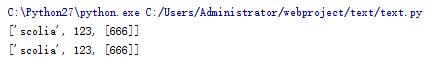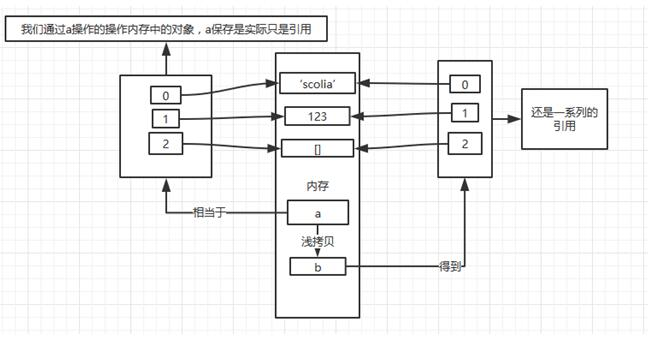```a = ['scolia', 123, [], ]
b = a[:]
print id(a), id(a), id(a), id(a)
print id(b), id(b), id(b), id(b)```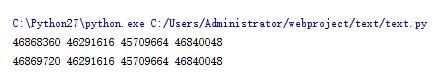```a = ['scolia', 123, [], ]
b = a[:]
b = 666
print a
print b```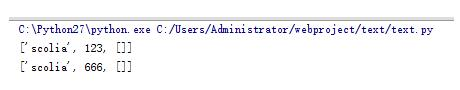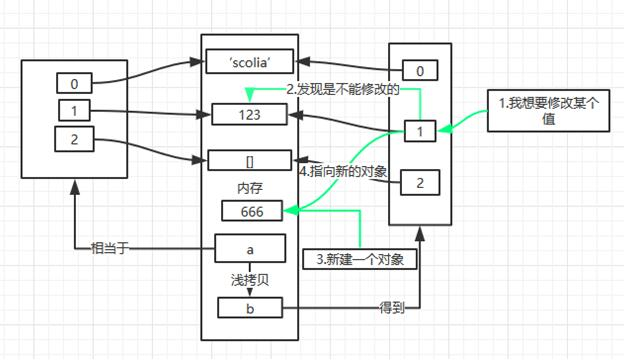```a = ['scolia', 123, [], ]
b = a[:]
b = 666
print id(a), id(a), id(a), id(a)
print id(b), id(b), id(b), id(b)```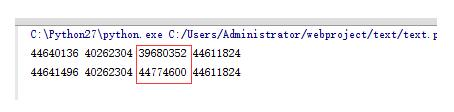1.切片操作

2.使用list()工厂函数新建对象。（ b = list(a) ）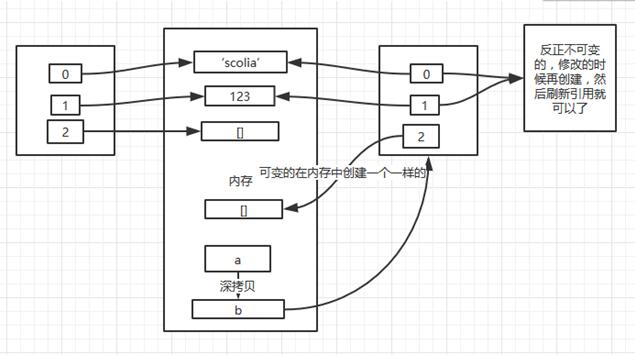```from copy import deepcopy
a = ['scolia', 123, [], ]
b = deepcopy(a)
b = 666
print id(a), id(a), id(a), id(a)
print id(b), id(b), id(b), id(b)```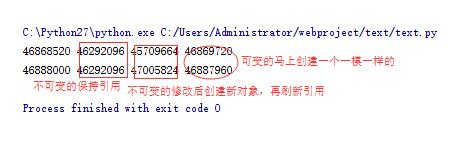1.正如代码示例用一样，只能通过内置的copy模块的deepcopy()方法创建。